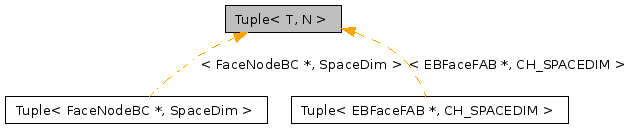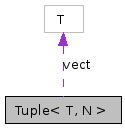# Tuple< T, N > Class Template Reference

`#include <Tuple.H>`

Inheritance diagram for Tuple< T, N >:[legend]
Collaboration diagram for Tuple< T, N >:[legend]

## Detailed Description

### template<class T, size_t N> class Tuple< T, N >

Ordered Tuples for Types T.

This class represents ordered tuples of some user-specified concrete type T for N > 0. The type T must have a default constructor. If the non-default constructor, copy constructor, or copy assignment operator are used, T must also have a copy constructor.

## Public Member Functions

Tuple ()
Tuple (const Tuple &rhs)
Tupleoperator= (const Tuple &rhs)
T & operator[] (int i)
const T & operator[] (int i) const
operator const T * () const

vect [N]

## Constructor & Destructor Documentation

template<class T, size_t N>
 Tuple< T, N >::Tuple ( ) ` [inline]`

: The default constructor. For user-defined types T, the default constructor for T will be run on each of the N objects in the Tuple. For builtin (intrinsic) types, the values in the Tuple will be garbage.

template<class T, size_t N>
 Tuple< T, N >::Tuple ( const Tuple< T, N > & rhs ) ` [inline]`

: Constructs a Tuple, initializing the elements in the Tuple with the corresponding elements in the vector v. This assumes that v contains at least N elements of type T -- an assumption that is NOT checked. For user-defined types, T must have a well-defined and accessible copy constructor.

References i, and Tuple< T, N >::vect.

## Member Function Documentation

template<class T, size_t N>
 Tuple< T, N > & Tuple< T, N >::operator= ( const Tuple< T, N > & rhs ) ` [inline]`

References i, and Tuple< T, N >::vect.

template<class T, size_t N>
 T & Tuple< T, N >::operator[] ( int i ) ` [inline]`

: Returns a modifiable lvalue reference to the i'th element in the Tuple, counting from zero. Performs range checking when the library is compiled in debug mode.

References Tuple< T, N >::vect.

template<class T, size_t N>
 const T & Tuple< T, N >::operator[] ( int i ) const` [inline]`

: Returns a constant reference to the i'th element in the Tuple, counting from zero. Performs range checking when the library is compiled in debug mode.

References Tuple< T, N >::vect.

template<class T, size_t N>
 Tuple< T, N >::operator const T * ( ) const` [inline]`

: Returns the address of the underlying vector of T representation. This should ONLY be used when interfacing to Fortran as it breaks the encapsulation of the class.

References Tuple< T, N >::vect.

## Member Data Documentation

template<class T, size_t N>
 T Tuple< T, N >::vect[N]` [protected]`

The documentation for this class was generated from the following file:

Generated on Tue Apr 14 14:24:02 2009 for Chombo + EB by1.5.5